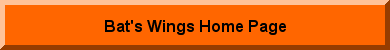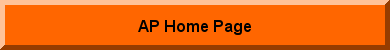Chapter 4  -  Ex 1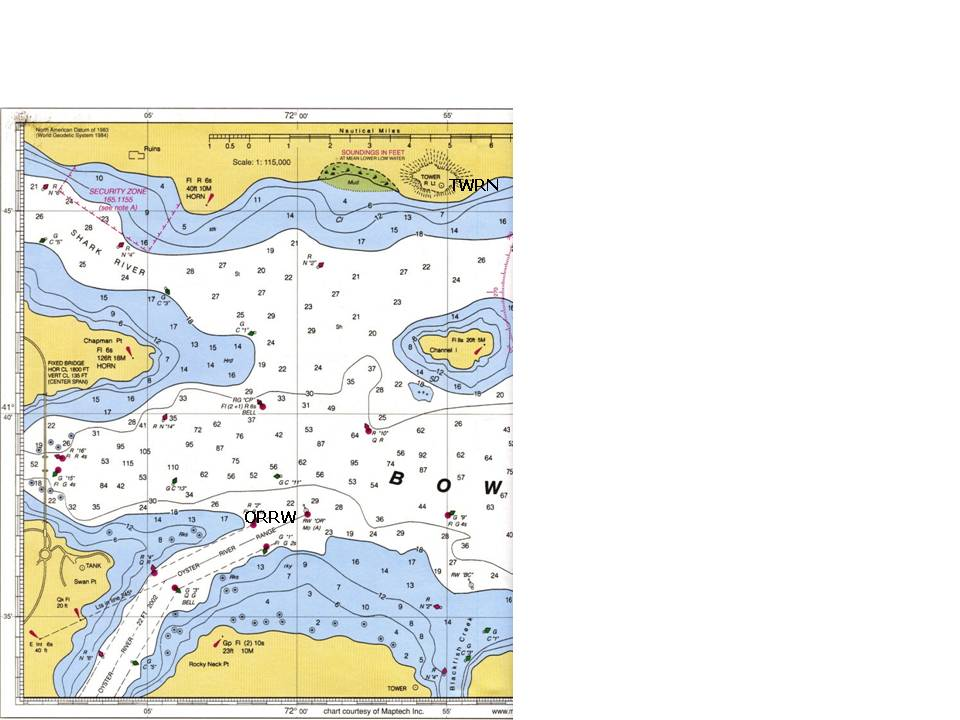Refer to plotting standards, p 124

Take out Bowditch Bay Chart.
Plan and plot a danger bearing using a visible landmark and monitoring the bearing to that landmark on the GPS screen.

Establish and plot a waypoint “TWRN” at Tower R Lt north of Channel Island. Assume that this tower is 40 feet high. Establish and plot a waypoint “ORRW” at RW “OR” Mo “A” Oyster River.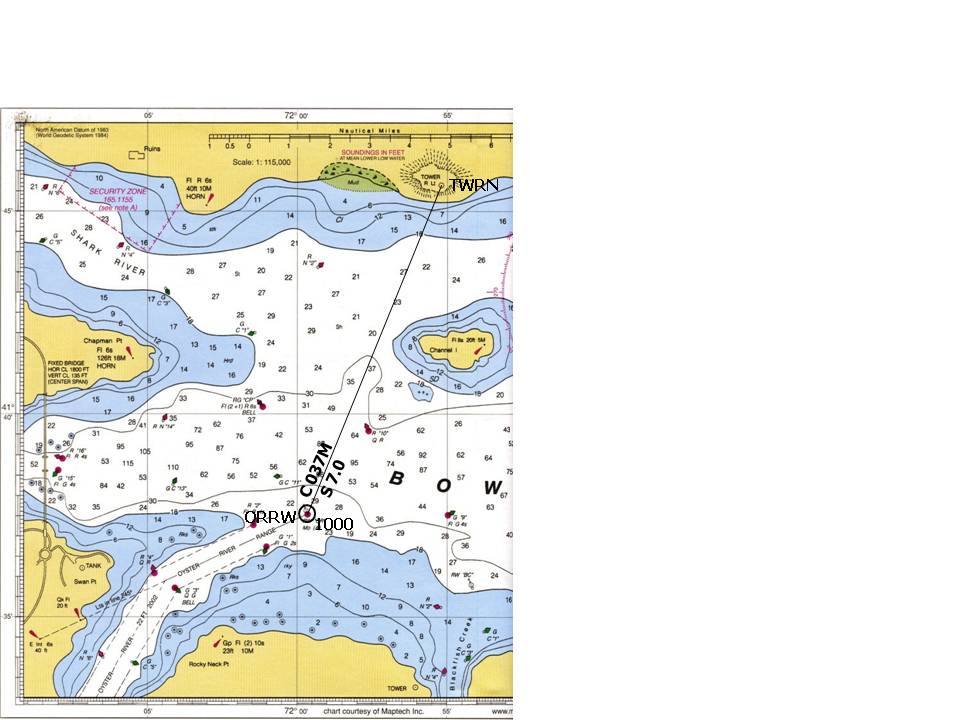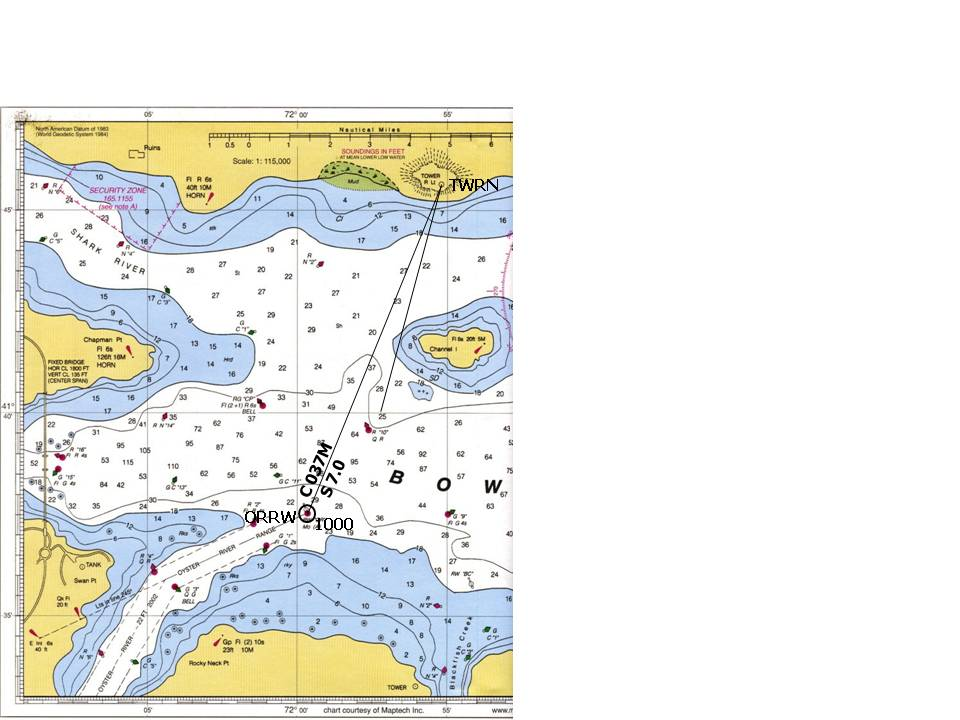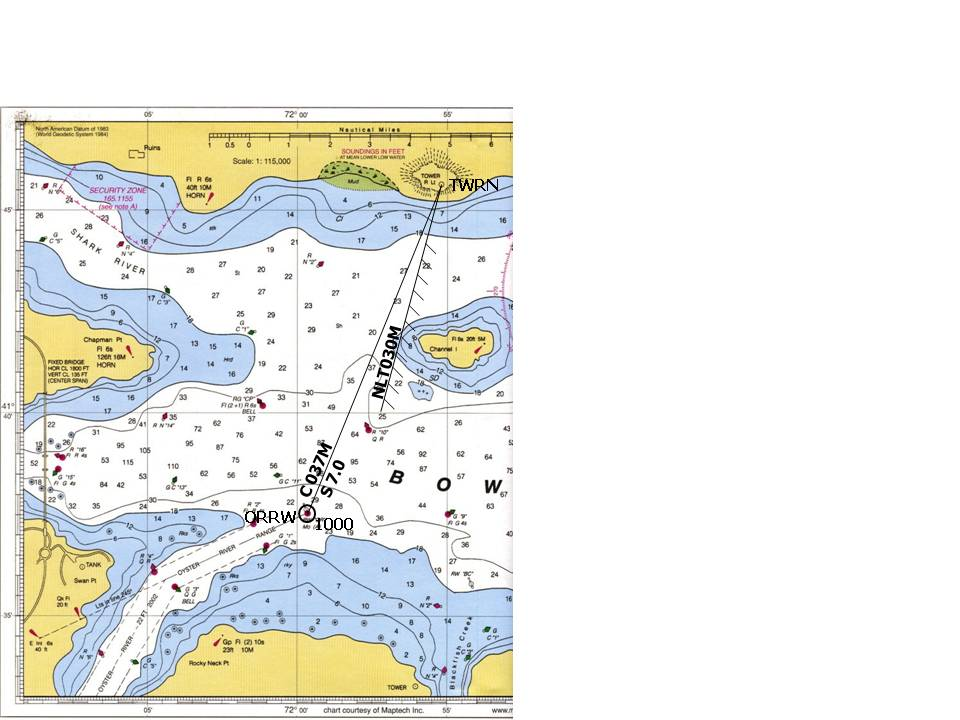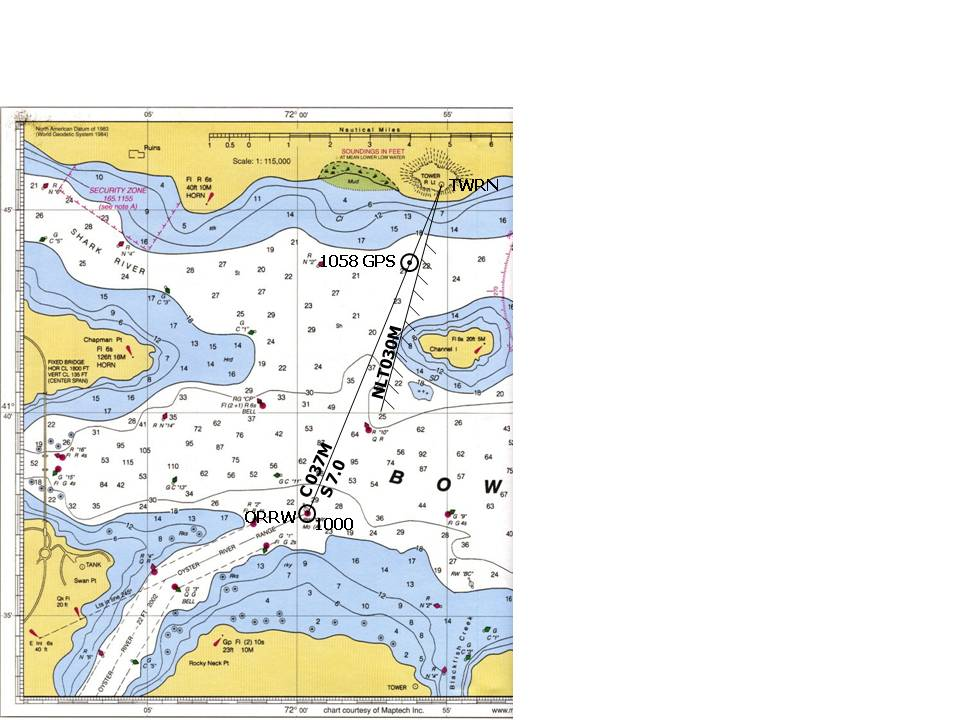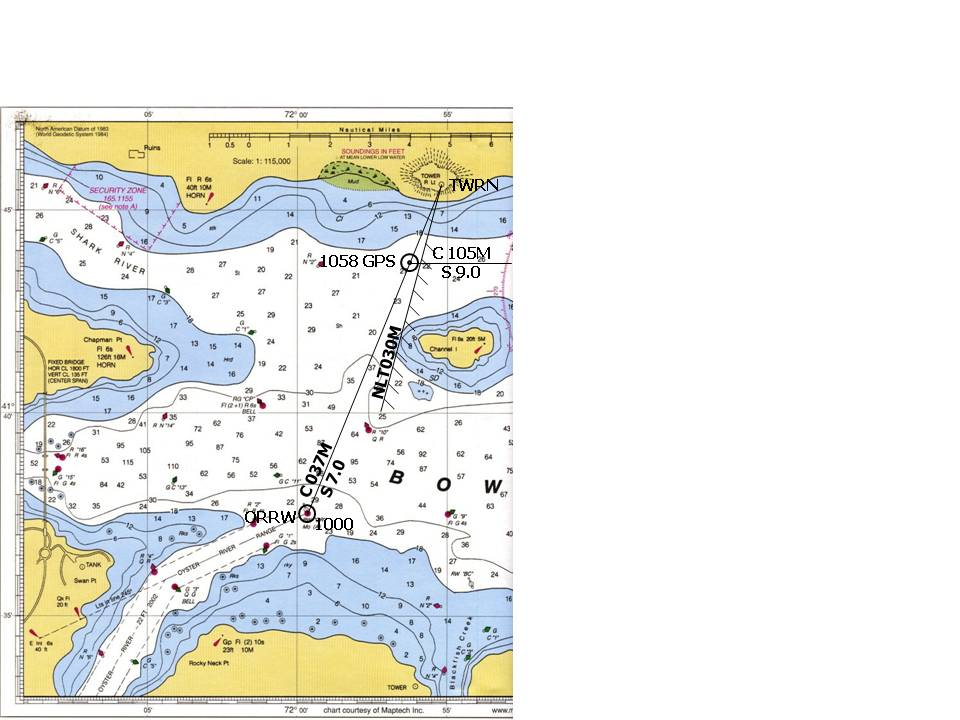At 1000, you depart waypoint ORRW. “Go To” TWRN. Your speed is 7.0kn.

T =022
V =015W
M = 037
D =000
C =037

Plot and label the course.

Plot a GPS danger bearing that will keep you west of Channel Island, coming no closer than the 12 foot sounding curve.

T =015
V =015W
M =     030
D =000
C =030

Draw the danger bearing
Determine the bearing of the danger bearing.

T =015
V =015W
M =      030
D =000
C =030

Draw and label the danger bearing – is it “not less than” or “not greater than”

From this fix, take up a magnetic heading of 105 and increase speed to 9.0kn.

M =105
V =015W
T =090

You always start a new course line from a fix.
At 1058, take a fix from your GPS screen when you reach a point 2.1nm from TWRN, with the waypoint bearing 037M.

T =022
V =015W
M =     037
D =000
C =037